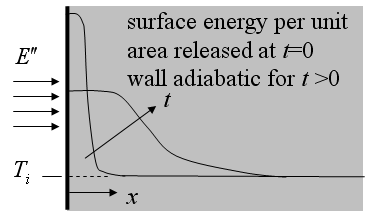Contents - Index

Energy PulseFunction semiinf4(T_i,E``,rho,c,alpha,x,time) returns the temperature for a given position and time within a semi-infinite body exposed to an impulse of energy per unit area at the surface using the relation provided in Table 3-2 of Nellis and Klein.

Inputs:
T_i - initial temperature of the solid  [C] or [K]
E`` - energy per unit area of impulse [J/m^2]
rho - density of solid [kg/m^3]
c - specific heat of solid [J/kg-K]
alpha - thermal diffusivity [m^2/s]
x - perpendicular distance from surface [m]
time - time relative to impulse (energy impulse occurs at t=0) [s]

This function can be used with English units set in EES.  In this case, T_i  is in [F] or [R],  E` is in Btu/ft^2, ,rho is in ft^2/lb_m, c is in Btu/lb_m-R, alpha is in ft^2/hr and x is in [ft].  Time must be in s.

Example:
\$UnitSystem SI K Pa J
T_i=393[K]
E``=14000 [J/m^2]
rho=8891 [kg/m^3]
c=750 [J/kg-K]
alpha=0.000001[m^2/s]
x=0.0005 [m]
time=8 [s]
T=semiinf4(T_i,E``,rho,c,alpha,x,time)

{Solution: T=393.4 [K]}

Transient Conduction Index